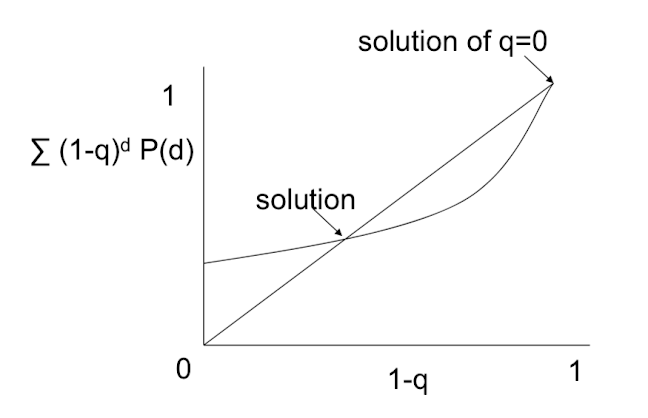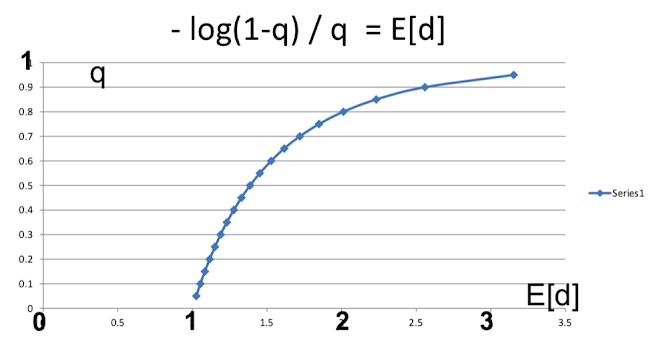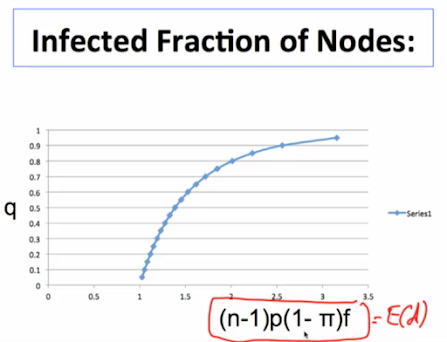# Calculating the Size of the Giant Component¶

• q is fraction of nodes in largest component

• look at any node: chance it is in the giant component is q

• chance that this node is outside of the giant component is the chance that all of its neighbors are outside of the giant component

• Probability that a node is outside of the giant component = 1-q

• = probability that all of its neighbors are outside
• = $(1-q)^d$ where d is the node's degree

# Giant Component Size¶

• Probability 1-q that a node is outside of the giant component is:

• $1-q = \sum_d (1-q)^dP(d)$
• where P(d) is the chance that the node has d neighbors
• Solve fo q...

It's a heuristic calculation because I'm not worrying about correlations and some of these variables I'm treating this as if they're independent. They're not quite independent. You can do a more exact calculation using generating functions. There's some of that in the book, but this will give us a fairly good approximation and work fairly well especially on large networks## Example¶# Giant Component Size: Poission Case¶

Solve $1-q = \sum_d(1-q)^dP(d)$

plug in a poisson distribution for PFD: $P(d) = [(n-1)^d/d!]p^d e^{-(n-1)p}$

so $1-q = e^{-(n-1)p} \sum_d[(1-q)(n-1)p]^d/d!$

## Useful Approximations¶

Taylor series approximation:

$e^x = 1 + x + \frac{x^2}{2!} + \frac{x^3}{3!}...$

$= \sum \frac{x^d}{d!}$

$[f(x) = f(a) + \frac{f'(a)(x-a)}{1!} + \frac{f''(a)(x-a)^2}{2!}...]$

so $1-q = e^{-(n-1)p} \sum_d[(1-q)(n-1)p]^d/d!$

$= e^{-(n-1)p}e^{(n-1)p(1-q)}$

$= e^{-q(n-1)p}$

$(n-1)p$ is the expected degree, p is the probability of any link

so $1-q = e^{-qE(d)}$

or $-\frac{log(1-q)}{q} = (n-1)p = E[d]$We get these Tyche phase transitions, so if expected neighbors less than "1" expected interactions that would transmit a diffusion of less than "1", we don't get diffusion. Once you hit one we get a diffusion and then it grows fairly rapidly and by the time you have three interactions then we're already to the point where you're very likely to hit most of the population. So if you remember in our promo video I said tell at least two friends. Well, if you tell just one friend about this then we don't get much diffusion. Two friends is at least enough to start pushing us fairly far along this. And you get to three friends and you're most of the way there.

So just in terms of giant component size here we are. We see that there's a fairly tight transition from having no giant component to having almost everybody being connected and so most of the play is in this one region. And indeed when people study epidemiology there's this idea that having at least one contact that's going to transmit something if people are doing more than one you're going to get diffusion or contagions and if people are having less than one transmission then things are going to die out. And that makes perfect sense. You need at least, you know, more than one and it's growing, fewer than one and it's shrinking. That's the critical point. And then here we find that it actually transitions very rapidly so that by the time you've got three interactions that are likely to lead to contagion then our diffusion is quite extensive.

# Who is infected?¶

• Probability of being in the giant component

• $1-(1-q)^d$ increasing in d

• More connected, more likely to be infected

(more likely to infected at any point in time...)

# Extensions:¶

• Immunity: delete a fraction of nodes and study the giant component on remaining nodes

• Probabilistic infection

• Random infection: have some links fail, just lower p

# Contagion with Immunity and Link Failure¶

• Some node is initially exposed to infection

• $\pi$ of the nodes are immune naturally

• only some links result in contagion - fraction f

• What is the extent of the infection?

• Consider a random network on n nodes

• Delete fraction $\pi$ of the nodes

• Delete fraction f of the links

• If starts at a node in giant component of the remaining network, then the giant component of that network is the extent of the infection; otherwise negligible

• Let q be the fraction of nodes of the remaining network in its giant component

• $q(1-\pi)$ is the probability of a nontrivial contagion

• Conditional on a contagion it infects $q(1-\pi)$ of the original nodes

• q solves $-log(1-q)/q = (n-1)p(1-\pi)f$

• some of those neighbors are immune and then also scaling down by the "f", so need to take these two modulations into effect# Implications¶

• Infection can fail if $\pi$ is high enough or f or p are low enough

• High $\pi$ - immunization, low virulence

• Low f - low contagiousness

• Low p - low contact among population